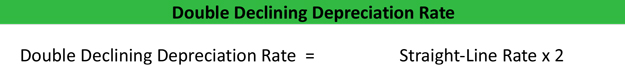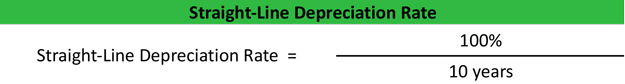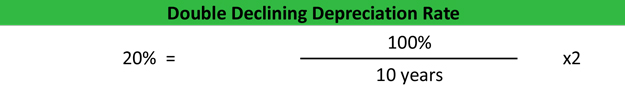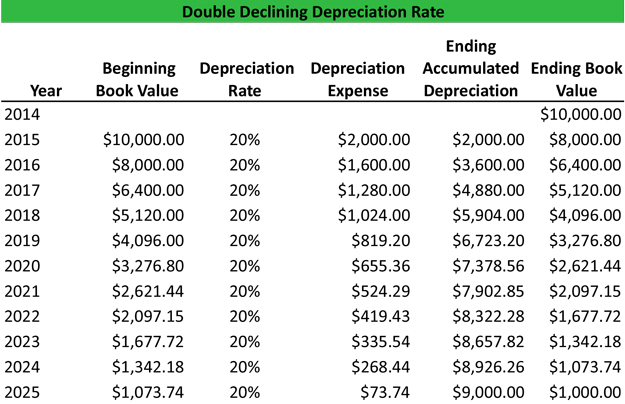# What is Double Declining Balance Depreciation (DDB)?

Definition: Double declining balance depreciation method is a form of allocating larger amounts of costs to an asset in earlier years of its useful life and lesser amounts in later years. In other words, it’s an accelerated depreciation method that frontloads the asset’s costs in the earlier years of its useful life.

## What Does Double Declining Balance Depreciation Mean?

The rationale behind the DDB method is that assets are more useful when they are new. Take a computer for instance. An average computer might last five years before it’s obsolete. The first two years of the computer’s life will be much more useful than the last two years because it is current and up to date when its new. The older the computer gets, the more sluggish and bogged down it becomes. Thus according to the matching principle, larger amounts of depreciation should be recognized in earlier years than later years.

The DDB method calculates depreciation for each period by multiplying the beginning book value by two times the straight-line method. Here is the double declining formula.As you can see the straight-line depreciation must be calculated before the DD method can be used. Let’s take a look at an example.

## Example

Assume a company purchases a piece of equipment with a 10-year useful life and a salvage value of \$1,000 for \$20,000. This is a ten-year asset, so the straight-line rate is calculated by dividing 100% by 10.The DD rate is twice the straight-line rate. Thus, the declining rate is 20 percent.Now it’s time for the actual calculation. The declining method multiplies the book value of the asset by the double declining depreciation rate. The depreciation expense is then recorded in the accumulated depreciation account, which reduces the asset book value for the next year. This happens every year of the asset’s useful life. Here is a spreadsheet example.Download this accounting example in excel to help calculate your own Double Declining Depreciation problems.